### Home > CALC > Chapter 3 > Lesson 3.1.1 > Problem3-15

3-15.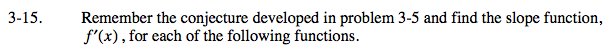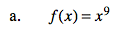BASIC CONJECTURE that you should have made during class:The slope function is one-degree lower than the original function.

ADVANCED CONJECTURE that you might have made during class:The slope function is one degree lower than the original function AND stretched by the degree of the original function.

slope function = 9x8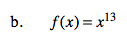Refer to hint for part (a).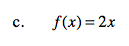This one does not require a conjecture - an Algebra I student could do it! This is the equation of a straight line. What is its slope?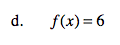Refer to hint for part (c).